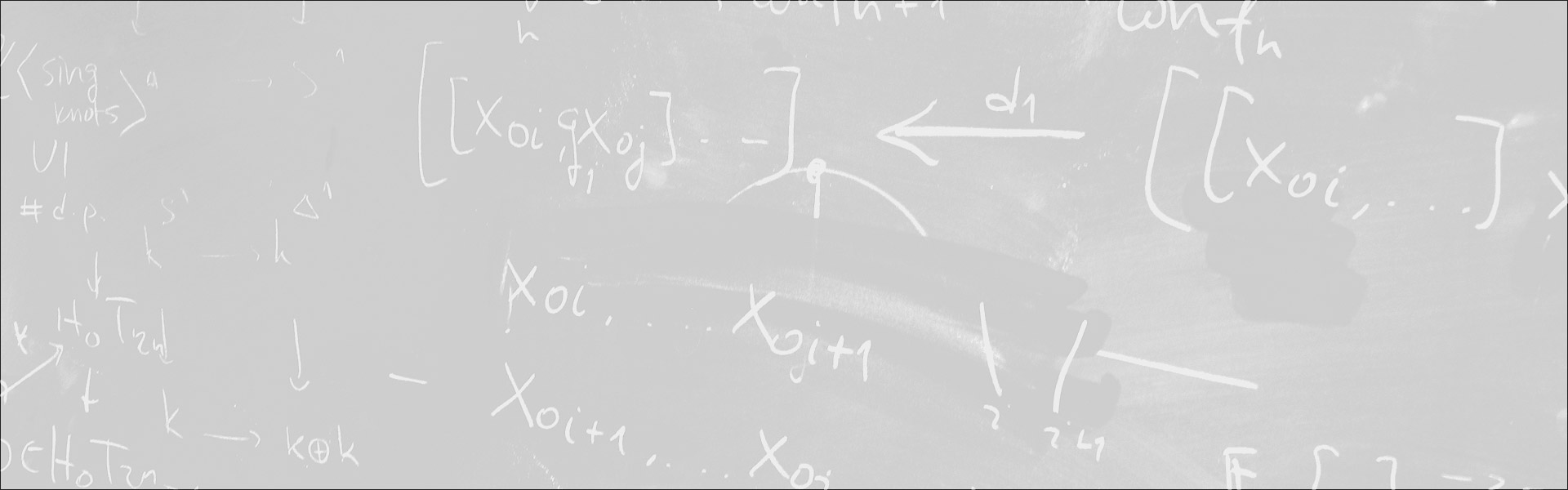Designed and built with care, filled with creative elements

Top# The dynamical Mordell-Lang problem in positive characteristic

/  Évènements• Cet évènement est passé

## The dynamical Mordell-Lang problem in positive characteristic

The dynamical Mordell-Lang conjecture in characteristic zero predicts that if f : X –> X is a map of algebraic varieties over a field K of characteristic zero, Y subset X is a closed subvariety and a in X(K) is a K-rational point on X, then the return set { n in N : f^n(a) in Y(K) } is a finite union of points and arithmetic progressions. For K a field of characteristic p > 0, it is necessary to allow for finite unions with sets of the form { a + sum_{i=1}^m p^{n_i} : (n_1, … , n_m) in N^m } and one might conjecture that all return sets are finite unions of points, arithmetic progressions and such p-sets. We studied the special case of the positive characteristic dynamical Mordell-Lang problem on semiabelian varieites and using our earlier results with Moosa on so-called F-sets reduced the problem to that of solving a class of exponential diophantine equations in characteristic zero. In so doing, under the hypothesis that X is a semiabelian variety and either Y has small dimension or f is sufficiently general, we prove the conjecture. However, we also show that our reduction to the exponential diiophantine problems may be reversed so that the positive characteristic dynamical Mordell-Lang conjecture in general is equivalent to a class of hard exponential diophantine problems which the experts consider to be out of reach given our present techniques. (This is a report on joint work with Pietro Corvaja, Dragos Ghioca and Umberto Zannier available at arXiv:1802.05309.)

- Séminaire Géométrie et théorie des modèles

#### Détails :

Orateur / Oratrice : Tom Scanlon
Date : 23 mars 2018
Horaire : 14h15 - 15h45
Lieu : IHP amphitheatre Darboux